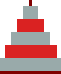## The shortest and "mysterious" TH algorithm AddendumM. Kolar
December 12, 2000

------ On Dec. 10, 2000, Glenn C. Rhoads wrote:

Yes, I'm already aware of that (one can get from the bits of the move count also which disk is being moved).

I've had the following (somewhat obfuscated) version up on my snippets page for some time (the code is supposed to resemble three disks stacked on top of each other):

 ``` main( ){int z,y,n ;scanf("%d",&n); for(y=1;(1<>=1);} ```
The original unobfuscated version is as follows:
 ```#include #include #define to(x) ((x|x-1)+1)%3 #define from(x) (x&x-1)%3 int disk(int); /* prototype */ void main( int argc, char *argv[] ) { int num_disks = atoi( argv ), move, max; max = (1 << num_disks); for (move=1; move < max; move++) printf("move %i: disk %i from pole %i to pole %i.\n", move, disk(move), from(move), to(move) ); } int disk(int move) { int ring=1; for( ;!(move&1); move >>= 1) ring++; return ring; }```
------ End of G.C. Rhoads' text

That tower-shaped snippet can be reached from http://remus.rutgers.edu/~rhoads/Code/code.html - a page full of wonderful C code - look for 'Hanoi' (and more can be found on http://remus.rutgers.edu/~rhoads/Obfuscated_C/obfuscate.html, e.g. http://remus.rutgers.edu/~rhoads/Obfuscated_C/pi.c - an amazing program to calculate Pi).
Dec. 2013: Since 2000, all these codes has moved to http://gcrhoads.byethost4.com/Code/code.html.

The tower-shaped snippet can actually be unfolded into a more usual form as follows:

 ```main(){ int z,y,n; scanf("%d",&n); for(y=1; (1<>=1) ; }```

This corresponds to replacing in my hBits.c code the inner for loop

 for(i=x, j=1; ; i>>=1, j++) { if(i&1) break; }
by this more compact statement
 for(i=x, j=1; !(i&1); i>>=1, j++) ;
However, this replacement had no noticeable effect on the CPU times for n=29.